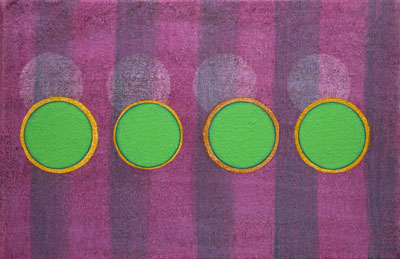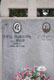Teresa Tramontina (1877-1928) 2008, oil/canvas, 20×30 cm queen of the night

 Fractional Diffusion and Fractional Calculus of Teresa Tramontina (1877-1928) - Walter Wyss; The fractional diffusion equation, Journal of Mathematical Physics 27, 1986. - Ben O'Shaughnessy and Itamar Procaccia; Analytical solutions for diffusion on fractal objects. Physics Review Letters 54, 1985. - Walter Wyss; Fractional diffusion and wave equations, Journal of Mathematical Physics 30, 1989. - Ralf Metzler, Walter Glöckle and Theo Nonnenmacher; Fractional model equation for anomalous diffusion, Physica 13, 1994. - Albert Compte; Stochastic foundations of fractional dynamics, Physical Review 53, 1996. - Bruce West, Paolo Grigolini, Ralf Metzler and Theo Nonnenmacher; Fractional diffusion and Lévy stable processes, Physical Review 55, 1997. - Theo Nonnenmacher; On the Riemann-Liouville fractional calculus and some recent applications. Fractals 3, 1995. - Keith Oldham and Jerome Spanier; The Fractional Calculus. Academic Press, New York, 1974. - Kenneth Miller and Bertram Ross; An Introduction to the Fractional Calculus and Fractional Differential Equations, John Wiley & Sons, New York, 1993. - Bruce West and William Deering; Fractal physiology for physicists: Lévy statistics, Physics Reports 246, 1994. - Gerd Baumann; Symmetry Analysis of Differential Equations Using Mathematica, Springer-Verlag, New York, 2000. - Hans Wilhelm Geiger and Ernest Marsden; The laws of deflexion of a particles through large angles, Philosophical Magazine 25, 1913. - Vladimir Aleksandrovich Marchenko; On the reconstruction of the potential energy from phases of the scattered waves, Dokl. Akad. Nauk SSSR, 1955. - Robert Miura, Clifford Gardner and Martin Kruskal; Korteweg-de Vries equation and generalizations, II. Existence of conservation laws and constants of motion, Journal of Mathematical Physics 9, 1968. - Thiab Taha and Mark Ablowitz; Analytical and numerical solutions of certain nonlinear evolution equations, Analytical. Journal of Computational Physics 55, 1984. - Hans Dehmelt; Radiofrequency Spectroscopy of stored ions I: Storage, Advances in atomic and molecular physics 53, 1967. - Hans Dehmelt; Monoelectron oscillator, Physics Review Letters 31, 1973. - Theo Nonnenmacher, Gerd Baumann and Gabriele Losa; Self organization and fractal scaling patterns in biological systems. In: Trends in Biological Cybernetics, World Scientific, Singapore, 1990. - Amnon Aharony; Structure and perimeters of percolation clusters, Journal of Physics A: Mathematical and General 19, 1986. - Pierre-Gilles de Gennes; Percolation - a new unifying concept, Recherche 7, 1980. - Gerd Baumann; Symmetry Analysis of Differential Equations using Mathematica, New York, 2000. - Harvey Scher and Elliott Montroll; Anomalous transit-time dispersion in amorphous solids. Physical Review B 12, 1975. - Helmut Schiessel and Alexander Blumen; Mesoscopic pictures of the sol-gel transition: Ladder models and fractal networks, Macromolecules 28, 1995. bibliographies Fractional Diffusion and Fractional Calculus of Teresa Tramontina (1877-1928) mx